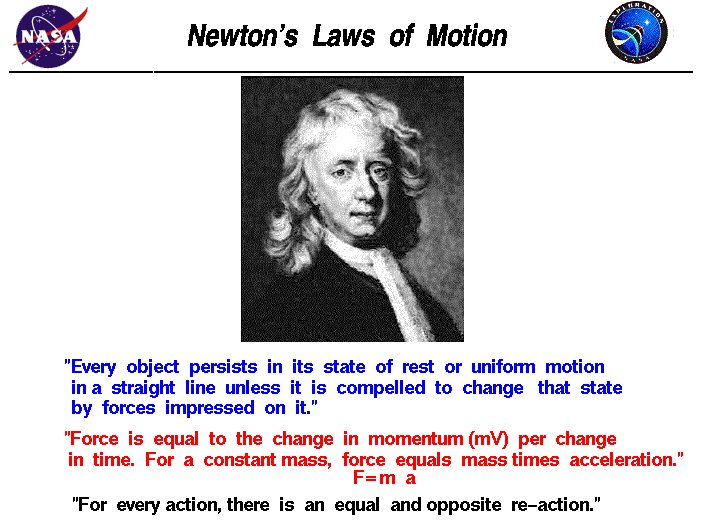+ Text Only Site
+ Non-Flash Version
+ Contact GlennThe motion of a rocket from the surface of the Earth to a landing on the Moon can be explained and described by physical principals discovered over 300 years ago by Sir Isaac Newton. Newton worked in many areas of mathematics and physics. He developed the theories of gravitation in 1666, when he was only 23 years old. Some twenty years later, in 1686, he presented his three laws of motion in the "Principia Mathematica Philosophiae Naturalis." The laws are shown above, and the application of these laws to rockets is given on separate slides. Newton's first law states that every object remains at rest or in uniform motion in a straight line unless compelled to change its state by the action of an external force. This is normally taken as the definition of inertia. The key point here is that if there is no net force acting on an object (if all the external forces cancel each other out) then the object maintains a constant velocity. If that velocity is zero, then the object remains at rest. If the velocity is not zero, then the object maintains that velocity and travels in a straight line. If a net external force is applied, the velocity changes because of the force. Velocity is a vector quantity, having both a magnitude and a direction. The change in velocity caused by a force may involve the magnitude, the direction, or both, depending on the magnitude and direction of the force, which is also a vector quantity. The second law explains how the velocity of an object changes when it is subjected to an external force. The law defines a force to be equal to change in momentum (mass times velocity) per change in time. Newton also developed the calculus of mathematics, and the "changes" expressed in the second law are most accurately defined in differential forms. Calculus can also be used to determine the velocity and location variations experienced by an object subjected to an external force. For an object with a constant mass m, the second law states that the force F is the product of an object's mass and its acceleration a: F = m * a For an object like a rocket, with a large change in mass during the flight, we must use the more accurate definition of the second law associated with the change in momentum. For an external applied force, the change in velocity depends on the mass of the object. A force causes a change in velocity; and likewise, a change in velocity generates a force. The equation works both ways. The third law states that for every action (force) in nature there is an equal and opposite re-action. In other words, if object A exerts a force on object B, then object B also exerts an equal force on object A. Notice that the forces are exerted on different objects. The third law can be used to explain the generation of thrust by a rocket engine. Guided ToursNewton's Laws of Motion:Activities: Fundamental Terminology: Grade 10-12 Hero Engine: Grade 6-10 Related Sites: Rocket Index Rocket Home Beginner's Guide Home+ Inspector General Hotline + Equal Employment Opportunity Data Posted Pursuant to the No Fear Act + Budgets, Strategic Plans and Accountability Reports + Freedom of Information Act + The President's Management Agenda + NASA Privacy Statement, Disclaimer, and Accessibility CertificationEditor: Tom Benson NASA Official: Tom Benson Last Updated: Jun 12 2014 + Contact Glenn